#### You may also likeA quadrilateral changes shape with the edge lengths constant. Show the scalar product of the diagonals is constant. If the diagonals are perpendicular in one position are they always perpendicular?### A Knight's Journey

This article looks at knight's moves on a chess board and introduces you to the idea of vectors and vector addition.### Which Twin Is Older?

A simplified account of special relativity and the twins paradox.

# 8 Methods for Three by One

##### Age 14 to 18Challenge Level
Update: Nov 2012: A new pure geometry method was sent in by Sigi. You can see Sigi's method here

Sigi suggests that certain solutions are dependent in that they use different notations for the same underlying contexts. Sigi suggests the following interesting task: Which of the different methods are genuinely mathematically independent of each other? Certainly something to think about.

Consider the problem is as follows: for the following diagram, prove that $a+b=c$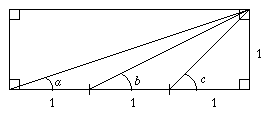This problem has been shown on NRICH before. When first shown on NRICH it was solved in 8 different ways by a pair of students Alex and Neil. When we again showed the problem, Sigi sent us a lovely geometric proof.

Once you have gone as far as you can with this, try to follow some of Alex's and Neil's solutions for the parts of mathematics with which you are most familiar:

Sigi's proof is three-by-one.pdf . The distinct proofs from Alex and Neil are:

Method 1: Tan Angle Sum Formula

Proof
From the diagram, $a =\tan^{-1}(1/3), b =\tan^{-1}(1/2)$, and $c = \tan^{-1}(1)$.

We have to prove $a+b=c$, which is the same as proving that $\tan^{-1}(1/3)+\tan^{-1}(1/2)=\tan^{-1}(1)$.

Note that
$$\begin{eqnarray} \tan\left(\tan^{-1}\left(\frac{1}{3}\right)+\tan^{-1}\left(\frac{1}{2}\right)\right) &=&\frac{\tan\left(\tan^{-1}\left(\frac{1}{3}\right)\right)+\tan\left(\tan^{-1}\left(\frac{1}{2}\right)\right)} {1-\tan\left(\tan^{-1}\left(\frac{1}{3}\right)\right)\tan\left(\tan^{-1}\left(\frac{1}{2}\right)\right)}\cr &=&\frac{\frac{1}{3}+\frac{1}{2}}{1-\frac{1}{3}\cdot\frac{1}{2}}\cr &=&1 \end{eqnarray}$$

Method 2: Sin Angle Sum Formula

Proof

Using Pythagoras we can calculate the lengths of the diagonal lines: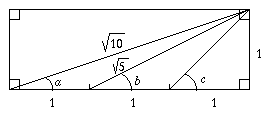$$\sin a = \frac{1}{\sqrt{10}}\quad \cos a = \frac{3}{\sqrt{10}}\quad \sin c = \frac{1}{\sqrt{2}}\quad \sin b = \frac{1}{\sqrt{5}}\quad \cos b = \frac{2}{\sqrt{5}}$$

Using the identity $\sin(a+b) = \sin a \cos b + \sin b \cos a$ we see that
$$\begin{eqnarray} \sin(a+b) &=& \frac{1}{\sqrt{10}}\cdot\frac{2}{\sqrt{5}}+\frac{1}{\sqrt{5}}\cdot \frac{3}{\sqrt{10}}\cr &=& \frac{2+3}{\sqrt{10\cdot 5}}\cr &=& \frac{5}{\sqrt{50}}\cr &=&\frac{1}{\sqrt{2}}\cr &=& \sin c \end{eqnarray}$$
Hence the result is proved.

Method 3: Cosine Rule

This method required us to extend the diagram as follows: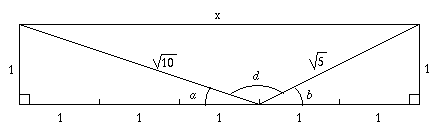From the altered diagram it can be seen that $x=3+2=5$.

Next, $d$ can be found using the cosine rule $c^2= a^2+b^2-2ab \cos C$.

Substitution of these values gives
$$\cos d = \frac{-1}{\sqrt{2}}$$
Thus $d=135^\circ$. Therefore $a+b = 180^\circ - d = 45^\circ = c$

Hence the result is proved.

Method 4: Vector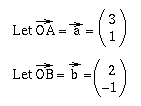Hence the result is proved.

Method 5: Matrices

Let $\bf{d} = (x, y)$ be any point in the $x-y$ plane. Let $\bf{d}_1$ be the point obtained by rotating $a^\circ$ about the origin.Let $\bf{d}_2$ be the point obtained by rotating $\bf{d}_1$ by $b^\circ$ around the origin.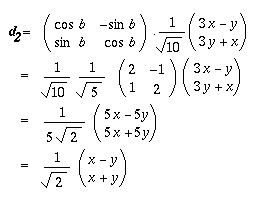Finally, let $\bf{d}_2$ be the point obtained by rotating $\bf{d}$ by $c^\circ$ around the origin.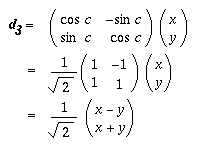Hence a rotation by $a+b$ is the same as a rotation by $c$ degrees. Hence, $a+b=c$, as none of the angles is greater than $90^\circ$.

Method 6: Pure Geometry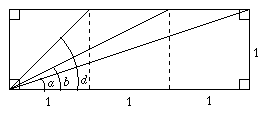By looking at the leftmost unit square this diagram can be drawn$a+b=c \Leftrightarrow x+x+y = x+y+z \Leftrightarrow x=z$
Hence it must be proven than $x=z$: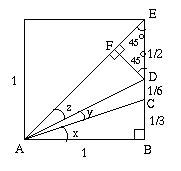From this it can be seen that
$$EF = DF = \frac{\sqrt{2}}{4}$$
and
$$AF = AE-FE = \sqrt{2} -\frac{\sqrt{2}}{4} = \frac{3\sqrt{2}}{4}$$

Thus
$$AF:AB = \frac{3\sqrt{2}}{4}:1$$
and
$$DF:BC = \frac{\sqrt{2}}{4}:\frac{1}{3} = \frac{3\sqrt{2}}{4}:1$$Hence ADF and ABC are similar triangles and, therefore, $x=z$. Thus, $a+b=c$.

Method 7: Coordinate Geometry

The coordinate geometry proof is based on the following diagram; the gradients are easy to read off the original image.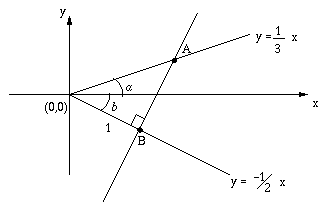Let $B$ be the point $( x_B ,y_B )$ on the line $y = -\frac{1}{2} x$  at a distance of $1$ from the origin. Since $\sqrt{x^2_B+y^2_B} = 1$ and $y_B = -\frac{1}{2}x_B$ we have
$$\begin{eqnarray} \sqrt{x^2_B+\frac{1}{4}x^2_B} &=& \sqrt{\frac{5}{4}x^2_B} = 1\cr \Rightarrow x_B = \frac{2}{\sqrt{5}}\cr \Leftrightarrow y_B = \frac{-1}{\sqrt{5}} \end{eqnarray}$$
Thus,
$$B=\left(\frac{2}{\sqrt{5}}, \frac{-1}{\sqrt{5}}\right)$$
We can now determine the equation of the line through $B$ perpendicular to the line $y = -\frac{1}{2} x$ to be
$$y = 2x-\sqrt{5}.$$
This line intersects $y=\frac{1}{3} x$ at $A$ which we can calculate to be
$$A= \left(\frac{3}{\sqrt{5}}, \frac{1}{\sqrt{5}}\right)$$
Now that we konw points $A$ and $B$ we can calculate the distance between them as
$$|AB| = \sqrt{\left(\frac{3}{\sqrt{5}}-\frac{2}{\sqrt{5}}\right)^2 +\left(\frac{1}{\sqrt{5}}+\frac{1}{\sqrt{5}}\right)^2}= \sqrt{\frac{1}{5}+\frac{4}{5}}=1$$
Referring back to the diagram shows that $a+b=45^\circ$ and the result is proved.

Method 8: Complex Numbers

This method uses aspects of other proofs presented (and therefore could be shortened) but we include it because it gives a nice example of how complex numbers, matrices and all other methods fit nicely together.

Let $r=\sqrt{x^2+y^2}$.

Now, the complex number corresponding to the point with coordinates $(x, y)$ in the argand diagram can be written as
$$z= x+iy = r\exp\left(i\tan^{-1} \left(\frac{y}{x}\right)\right)$$
Let $z'$ be the complex number obtained by rotating $z$ by $2(a+b)$ degrees. Then we can use the identities $\sin(a+b) = \sin a \cos b +\cos a\sin b$ and $\cos(a+b) = \cos a \cos b-\sin a \sin b$ to see that:
$$\begin{eqnarray} z' &=& r\exp\left(i\tan^{-1}\left(\frac{y}{x}\right)+2i(a+b)\right)\cr &=& r\left[\cos\left(\tan^{-1}\left(\frac{y}{x}\right)+2(a+b)\right)+i\sin\left(\tan^{-1}\left(\frac{y}{x}\right)+2(a+b)\right)\right]\cr &=&r\left[\cos\left(\tan^{-1}\left(\frac{y}{x}\right)\right)\cos\left(2(a+b)\right)-\sin\left(\tan^{-1}\left(\frac{y}{x}\right)\right)\sin\left(2(a+b)\right)\right]\cr && +ir\left[ \sin\left(\tan^{-1}\left(\frac{y}{x}\right)\right)\cos\left(2(a+b)\right)+\cos\left(\tan^{-1}\left(\frac{y}{x}\right)\right)\sin\left(2(a+b)\right) \right] \end{eqnarray}$$

We  can simplify the arctangents by considering the following diagram: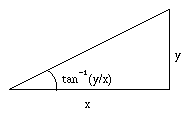From this we can see that $\cos\left(\tan^{-1}\left(\frac{y}{x}\right) \right)= \frac{x}{r}$ and $\sin\left(\tan^{-1}\left(\frac{y}{x}\right) \right)= \frac{y}{r}$
This simplifies the expression for $z'$ to
$$z' = x\cos\left(2(a+b)\right)-y\sin\left(2(a+b)\right)+i\left(y\cos\left(2(a+b)\right)+x\sin\left(2(a+b)\right)\right)$$

Alex and Neil then used some double angle formulae and the fact that $\sin(a+b)=\cos(a+b)=\frac{1}{\sqrt{2}}$ to determine that
$$z' = -y+ix$$
Since this is a complex number rotated through $90^\circ$ we can conclude that $a+b = 45^\circ$